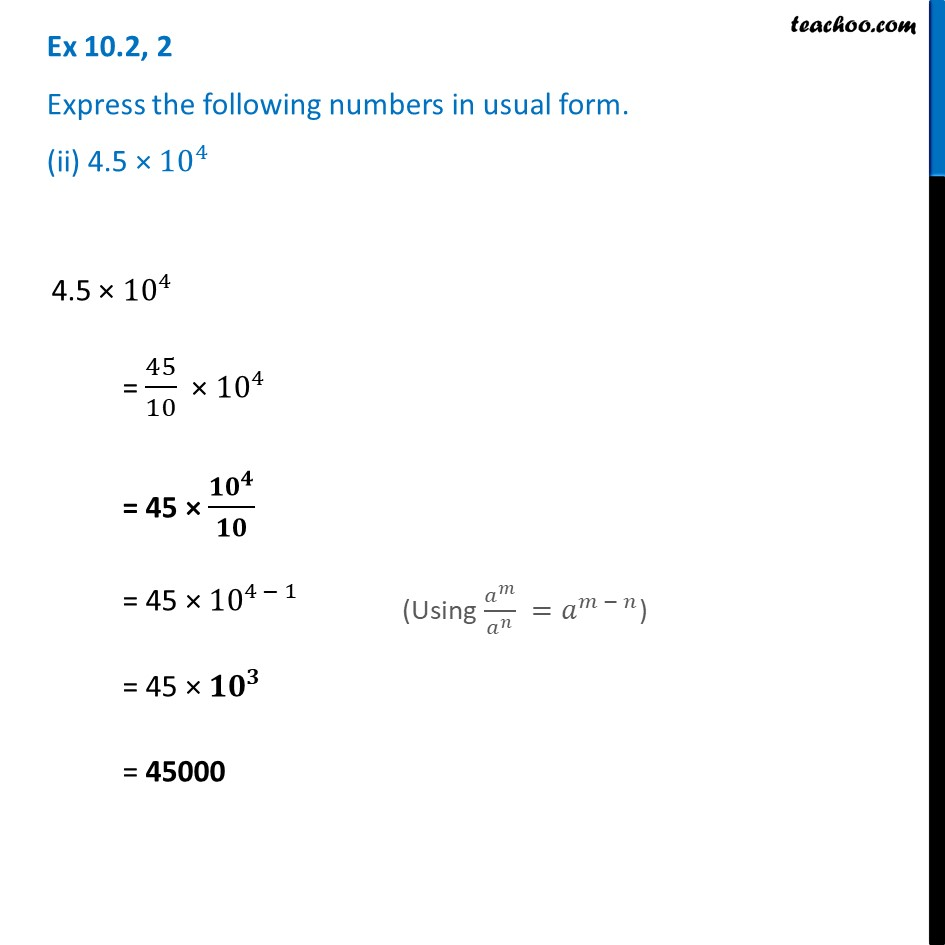Ex 10.2

Chapter 10 Class 8 Exponents and Powers
Serial order wiseLearn in your speed, with individual attention - Teachoo Maths 1-on-1 Class

### Transcript

Ex 10.2, 2 Express the following numbers in usual form. (ii) 4.5 × 10^44.5 × 10^4 = 45/10 × 10^4 = 45 × 〖𝟏𝟎〗^𝟒/𝟏𝟎 = 45 × 10^(4 − 1) = 45 × 〖𝟏𝟎〗^𝟑 = 45000